Test: Group Theory - 1

# Test: Group Theory - 1

Test Description

## 20 Questions MCQ Test Topic-wise Tests & Solved Examples for IIT JAM Mathematics | Test: Group Theory - 1

Test: Group Theory - 1 for Mathematics 2023 is part of Topic-wise Tests & Solved Examples for IIT JAM Mathematics preparation. The Test: Group Theory - 1 questions and answers have been prepared according to the Mathematics exam syllabus.The Test: Group Theory - 1 MCQs are made for Mathematics 2023 Exam. Find important definitions, questions, notes, meanings, examples, exercises, MCQs and online tests for Test: Group Theory - 1 below.
Solutions of Test: Group Theory - 1 questions in English are available as part of our Topic-wise Tests & Solved Examples for IIT JAM Mathematics for Mathematics & Test: Group Theory - 1 solutions in Hindi for Topic-wise Tests & Solved Examples for IIT JAM Mathematics course. Download more important topics, notes, lectures and mock test series for Mathematics Exam by signing up for free. Attempt Test: Group Theory - 1 | 20 questions in 60 minutes | Mock test for Mathematics preparation | Free important questions MCQ to study Topic-wise Tests & Solved Examples for IIT JAM Mathematics for Mathematics Exam | Download free PDF with solutions
 1 Crore+ students have signed up on EduRev. Have you?
*Multiple options can be correct
Test: Group Theory - 1 - Question 1

### Consider the following relations, which of the following is an equivalence relation?

Detailed Solution for Test: Group Theory - 1 - Question 1

The equivalence class ofunder ~, denoted, is defined asTest: Group Theory - 1 - Question 2

### If G is a Prime order group then G has

Detailed Solution for Test: Group Theory - 1 - Question 2

The order of subgroups divides the Order of Group but if some m divide the order of the group that doesn't mean that group has the subgroup of order m

Proper subgroup :  H ≠ e and H ≠ G is the subgroup of G

Calculations:

G is a Prime order Group of p

so, it has only 2 divisors 1 and itself that divides the order of Group

⇒ Only 2 subgroups are generated by 1 and p

⇒ <1> = G and

= {e} where e is the identity

∴ option 1 is correct, No proper Subgroup

Test: Group Theory - 1 - Question 3

### Let n be a fixed positive integer. Define a relation R on the set Z of integers by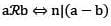then R is

Detailed Solution for Test: Group Theory - 1 - Question 3

Explanation : Reflexive: For any a∈N, we have a−a=0=0×n⇒−a is divisible by n

⇒(a,a)∈R

Thus (a,a)∈R for all a∈Z. So, R is reflexive.

Symmetry: Let (a,b)∈R. Then,

⇒(a,b)∈R

⇒(a−b) is divisible by n.

⇒(a−b)=np for some p∈Z

⇒b−a=n(−p)

⇒b−a is divisible by n

Thus, (a,b)∈R

⇒(b,a)∈R for all a,b∈Z

So, R is symmetric on Z.

Transitive: Let a,b,c∈Z such that (a,b)∈R and (b,c)∈R. Then (a,b)∈R

⇒(a−b) is divisible by n.

⇒a−b=np for some p∈Z

(b,c)∈R

⇒(b−c) is divisible by n.

⇒b−c=nq for some q∈Z

therefore,(a,b)∈R and b−c∈R

⇒a−b=npb−c=nq

⇒(a−b)+(b−c)=np+nq

a−c=n(p+q)

⇒−c is divisible by n.

⇒(a−c)∈R

Thus, (a,b)∈R and (b,c)∈R

⇒(a,c)∈R for all a,b,c∈Z

So, R is a transitive relation on Z.

Thus, R being reflexive, symmetric and transitive, is an equivalence relation.

Test: Group Theory - 1 - Question 4

Let R be a relation over the set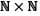and it is defined by (a, b) R (c, d) ⇔ a + d = b + c then R is

Detailed Solution for Test: Group Theory - 1 - Question 4

(a, b) R (c, d) ⇒ a + d = b + c

(a, a) R (a, a) ⇒ 2a = 2a

It is reflexive.

(a, b) R (c, d) ⇒ (c, d) R (a, b)

a + d = b + c

b + c = a + d

It is symmetric.

Transitive:

(a, b) R (c, d) ⇒ (c, d) R (e, f) ⇒ (a, b) R (e, f)

a + d = b + c, c + f = d + c

a – e = b – f

a + f = b + c

(a, b) R (e, f)

It is transitive.

Test: Group Theory - 1 - Question 5

Number of proper Subgroups of Zis

Detailed Solution for Test: Group Theory - 1 - Question 5

Concept used:

Improper subgroup means The subgroup is Group itself

Proper Subgroup of Zn = The subgroups which are not improper

Number of subgroups of Z = Number  of divisors of n

Calculations:

The number of divisors of 6 = 1, 2, 3, 6

Total subgroups is 4

but One subgroup is equal to Group that is improper subgroup generated by <1> = {0, 1, 2, 3, 4, 5}

∴ Total number of proper subgroup of Z = 3

*Multiple options can be correct
Test: Group Theory - 1 - Question 6

An integer m is said to be related to another integer n if m is a multiple of n then relation is

Detailed Solution for Test: Group Theory - 1 - Question 6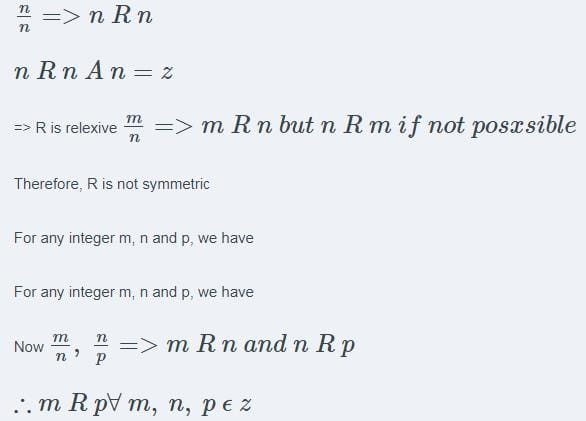*Multiple options can be correct
Test: Group Theory - 1 - Question 7

Which of the following statements is correct?

Detailed Solution for Test: Group Theory - 1 - Question 7

The empty relation is the subset  ∅. It is clearly irreflexive, hence not reflexive. To check symmetry, we want to know whether  aRb⇒bRa  for all  a,b∈A.

Test: Group Theory - 1 - Question 8

The number of elements Satisfies g7 = e, g ≠ e in any finite group

Detailed Solution for Test: Group Theory - 1 - Question 8

Concept used:

Order of generator = Order of group

Every element in the group must divide the order of group and satisfies the property d7 = e

Explanations:

g7 = e

o(g)\7

Two number divides 7 that is 1 or 7

Now,

g ≠ e

⇒ o(g) = 7

Let us consider that the subgroup generated by g.

As the order of g is 7

The order of the subgroup generated by g is 7 (because order of generator = order of group)

⇒ no of elements satisfies g= 7 is odd (every element in the group must divide the order of group and satisfies the property d7 = e}

∴ option 2 is correct

*Multiple options can be correct
Test: Group Theory - 1 - Question 9

The relation R defined on the set A ={1,2,3,4,5} by P = {(x, y) : |x2 —y2| < 16} is given by

Detailed Solution for Test: Group Theory - 1 - Question 9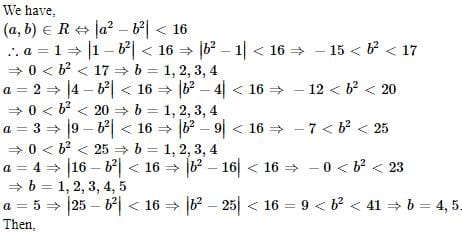R= {(1,1),(1,2),(1,3),(1,4),(2,1),(2,2),(2,3),(2,4),(3,1),(3,2),(3,3),(3,4),(4,1),(4,2),(4,3),(4,4),(4,5),(5,4),(5,5)}R={(1,1),(1,2),(1,3),(1,4),(2,1),(2,2),(2,3),(2,4),(3,1),(3,2),(3,3),(3,4),(4,1),(4,2),(4,3),(4,4),(4,5),(5,4),(5,5)}

Test: Group Theory - 1 - Question 10

Which of the following is not an equivalence relation in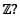Detailed Solution for Test: Group Theory - 1 - Question 10

As, a<b doesn't imply b<a ,which make the relation asymmetric and thus it is not an equivalence relation .

Test: Group Theory - 1 - Question 11

Let G be a finite group and Z10 be a homomorphic image of G. Then

Detailed Solution for Test: Group Theory - 1 - Question 11

Let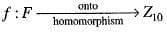So, Z10 is isomorphic to G/Ker f (by fundamental theorem of homomorphism)
implies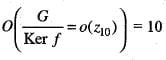implies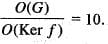Test: Group Theory - 1 - Question 12

The number of all subgroups of the group (Z60, +) of integers modulo 60 is

Detailed Solution for Test: Group Theory - 1 - Question 12

Group (Z60, +) of integer modulo 60.
Order of Subgroup will divide order of the group.
60 = 22 • 3 • 5
So, total number of divisor = 3 x 2 x 2 = 12
So, 12 subgroups are possible.
Since operation is addition modulo 60 thus each divisor will form a subgroup.
Thus, there are 12 subgroups.

Test: Group Theory - 1 - Question 13

Choose the correct option.

Detailed Solution for Test: Group Theory - 1 - Question 13

Remember: There is essentially only one cyclic group of each order (upto isomorphism).

Test: Group Theory - 1 - Question 14

Let G = { 1, 2,................, p-1} be the group with respect to multiplication modulo p. If the inverse of 110 in G is 4, then p is of the form

Detailed Solution for Test: Group Theory - 1 - Question 14

Let G = ( 1 , 2 , . . . , p - 1 } be the group w.r.t. multiplication modulo p.
Given, the inverse o f 110 is 4
i.e. 110 x 4 = 1 (mod p)
implies 440 = (1 mod p)
implies p = 440 - 1
p = 439 —> which is of the form of 5n+4

Test: Group Theory - 1 - Question 15

Consider the group 2 x 2 non-singular matrices under matrix multiplication over Z5. The inverse of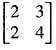is

Detailed Solution for Test: Group Theory - 1 - Question 15

Given the group of 2 x 2 non-singular matrices under matrix multiplication over Z5.
The inverse of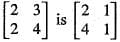i.e.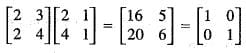over Z5.

Test: Group Theory - 1 - Question 16

a, b ε G, a group. If o(a) = 10 and o(b) = 21, then (Where <a> means cyclic group generated by a.)

Detailed Solution for Test: Group Theory - 1 - Question 16

[Remember o(H ∩ K) divides o(H) and o(K) where H and K are groups]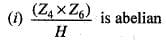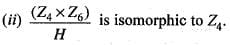Test: Group Theory - 1 - Question 17

Consider the following statements:
1. A group of order 289 is abelian.
2. In S3 there are four elements satisfying x2= e and six elements satisfying y3 = e.
3. Every proper subgroup of S3 is cyclic.
4. (Z30,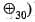has 4 subgroups of order 15 .
Correct statements are:

Detailed Solution for Test: Group Theory - 1 - Question 17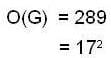every group of order p2 (p -> prime) is always abelian group.
(1) true

In S3 → total no. of elements are 3 of order 2 i.e. x2 = e and identity element also satisfying this condition total elements are 4. but only 3 elements are exists which satisfies the condition y3 = e.
(3) A3 is proper subgroup of S3 which is cyclic.

Test: Group Theory - 1 - Question 18

Which of the following group is cyclic ?

Detailed Solution for Test: Group Theory - 1 - Question 18

Concept used:

A group is cyclic iff there exists an element of order whose order is equal to the order of the group

Calculations:

o(S3) = 3!

contains 6 elements of the Symmetric group S(3), of degree 3, written in cycles are : {(1), (12), (13), (23), (123), (321)}.

o(1) = 1

o(12), o(23) and o(13) = 2

o(123),  and  o(321) = 3

no element having order equal to order of group

∴ option 1 is incorrect.

o(D4) = 8

D4 = {1, r, r2, r3 , s, sr, sr2, sr3}

o(s), o(sr), o(sr2), o(sr3) = 2

o(1) = 1, o(r) = 2 , o(r2) = 3, o(r3) = 4

no element having order equal to order of the group

∴ option 3 is incorrect.

o(K4) = 4

K4  = {e, a, b, ab}

o(e) = 1 , o(a) = 2, o(b) = 2, o(ab) = 2

No element having order equal to the order of group

∴ option 4 is incorrect.

o(Z5) = 5

Z5 = {0, 1, 2, 3, 4}

o(0) = 1 o(1) = 5, o(2) = 5 , o(3) = 5 , o(4) = 5

all elements having order = order of the group

all are the generator of the group.

∴ option 2 is correct.

Test: Group Theory - 1 - Question 19

Let G be a group order 6, and H be a subgroup of G such that 1 < |H| < 6. Which one of the following options is correct?

Detailed Solution for Test: Group Theory - 1 - Question 19

Concept

According to the Lagrange theorem order of subgroups must divide the order of the group.

Property of group says if a group has prime order then it is cyclic.

Explanation:

Since the order of G is 6. Therefore  its subgroup may have order 1,2,3,6

H is one of its subgroups with condition 1< |H| <6 so H may be of order 2 or 3  which is prime

Hence H must be cyclic

The order of G is 6 which is not prime and hence it may or may not  be cyclic

Therefore option 2 is correct

Test: Group Theory - 1 - Question 20

If (G, ⋅) is a group such that (ab)-1 = a-1 b-1, ∀ a, b ∈ G, then G is a/an

Detailed Solution for Test: Group Theory - 1 - Question 20

A group is said to be abelian if (a*b) = (b*a) ∀a,b ∈G

Since (G, ⋅) is a group

∴ (ab)-1 = (b-1a-1)             (1)

Given: (ab)–1 = a–1b–1       (2)

From (1) and (2)

(a*b) = (b*a)

Therefore it is abelian

## Topic-wise Tests & Solved Examples for IIT JAM Mathematics

27 docs|150 tests
 Use Code STAYHOME200 and get INR 200 additional OFF Use Coupon Code
Information about Test: Group Theory - 1 Page
In this test you can find the Exam questions for Test: Group Theory - 1 solved & explained in the simplest way possible. Besides giving Questions and answers for Test: Group Theory - 1, EduRev gives you an ample number of Online tests for practice

## Topic-wise Tests & Solved Examples for IIT JAM Mathematics

27 docs|150 tests